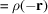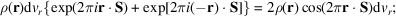International
Tables for
Crystallography
Volume F
Crystallography of biological macromolecules
Edited by E. Arnold, D. M. Himmel and M. G. Rossmann

International Tables for Crystallography (2012). Vol. F, ch. 2.1, pp. 54-55   | 1 | 2 |

Section 2.1.4.3. Scattering by atoms

J. Drentha*

aLaboratory of Biophysical Chemistry, University of Groningen, Nijenborgh 4, 9747 AG Groningen, The Netherlands
Correspondence e-mail: j.drenth@chem.rug.nl

| top | pdf |

2.1.4.3.1. Scattering by one atom

| top | pdf |

Electrons in an atom are bound by the nucleus and are – in principle – not free electrons.

However, to a good approximation, they can be regarded as such if the frequency of the incident radiation ν is greater than the natural absorption frequencies,, at the absorption edges of the scattering atom, or the wavelength of the incident radiation is shorter than the absorption-edge wavelength (Section 2.1.4.4). This is normally true for light atoms but not for heavy ones (Table 2.1.4.1).Table 2.1.4.1| top | pdf | The position of the Kα edge of different elements
Atomic numberElementKα edge (Å)
6 C 43.68
16 S 5.018
26 Fe 1.743
34 Se 0.980
78 Pt 0.158

If the electrons in an atom can be regarded as free electrons, the scattering amplitude of the atom is a real quantity, because the electron cloud has a centrosymmetric distribution, i.e..

A small volume,, at r containselectrons, and at −r there areelectrons. The combined scattering of the two volume elements, in units of the scattering of a free electron, isthis is a real quantity.

The scattering amplitude of an atom is called the atomic scattering factor f. It expresses the scattering of an atom in terms of the scattering of a single electron. f values are calculated for spherically averaged electron-density distributions and, therefore, do not depend on the scattering direction. They are tabulated in IT C (2004)as a function of. The f values decrease appreciably as a function of(Fig. 2.1.4.5).This is due to interference effects between the scattering from the electrons in the cloud. In the direction, all electrons scatter in phase and the atomic scattering factor is equal to the number of electrons in the atom.Figure 2.1.4.5 | top | pdf |The atomic scattering factor f for carbon as a function of, expressed in units of the scattering by one electron. Reproduced with permission from Drenth (1999). Copyright (1999) Springer-Verlag.

2.1.4.3.2. Scattering by a plane of atoms

| top | pdf |

A plane of atoms reflects an X-ray beam with a phase retardation ofwith respect to the scattering by a single atom. The difference is caused by the difference in path length from source (S) to atom (M) to detector (D) for the different atoms in the plane (Fig. 2.1.4.6).Suppose the plane is infinitely large. The shortest connection between S and D via the plane is SMD. The plane containing S, M and D is perpendicular to the reflecting plane, and the lines SM and MD form equal angles with the reflecting plane. Moving outwards from atom M in the reflecting plane, to P for instance, the path length SPD is longer. At the edge of the first Fresnel zone, the path islonger (Fig. 2.1.4.6).This edge is an ellipse with its centre at M and its major axis on the line of intersection between the plane SMD and the reflecting plane. Continuing outwards, many more elliptic Fresnel zones are formed. Clearly, the beams radiated by the many atoms in the plane interfere with each other. The situation is represented in the Argand diagram in Fig. 2.1.4.7.Successive Fresnel zones can be subdivided into an equal number of subzones. If the distribution of electrons is sufficiently homogeneous, it can be assumed that the subzones in one Fresnel zone give the same amplitude at D. Their phases are spaced at regular intervals and their vectors in the Argand diagram lie in a half circle. In the lower part of Fig. 2.1.4.7,this is illustrated for the first Fresnel zone. For the second Fresnel zone (upper part), the radius is slightly smaller, because the intensity radiated by more distant zones decreases (Kauzmann, 1957). Therefore, the sum of vectors pointing upwards is shorter than that of those pointing downwards, and the resulting scattered wave lagsin phase behind the scattering by the atom at M.Figure 2.1.4.6 | top | pdf |S is the X-ray source and D is the detector. The scattering is by the atoms in a plane. The shortest distance between S and D via a point in the plane is through M. Path lengths via points in the plane further out from M are longer, and when these beams reach the detector they lag behind in phase with respect to the MD beam. The plane is divided into zones, such that from one zone to the next the path difference is.Figure 2.1.4.7 | top | pdf |Schematic picture of the Argand diagram for the scattering by atoms in a plane. All electrons are considered free. The vector of the incident beam points to the left. The atom at M (see Fig. 2.1.4.6) has a phase difference of π with respect to the incident beam. Subzones in the first Fresnel zone have the endpoints of their vectors on the lower half circle. For the next Fresnel zone, they are on the upper half circle, which has a smaller radius because the amplitude decreases gradually for subsequent Fresnel zones (Kauzmann, 1957). The sum of all vectors points down, indicating a phase lag ofwith respect to the beam scattered by the atom at M.

References

International Tables for Crystallography (2004). Vol. C. Mathematical, Physical and Chemical Tables, edited by E. Prince. Dordrecht: Kluwer Academic Publishers.
Kauzmann, W. (1957). Quantum Chemistry. New York: Academic Press.